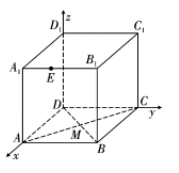$\text{A.}$ 存在点 $M$, 使得 $C_1 M+M E=5$ $\text{B.}$ 若 $M E / /$ 平面 $A_1 B D$ 时, $M E$ 长度的最小值是 $\sqrt{6}$ $\text{C.}$ 若 $A_1 M$ 与平面 $A B C D$ 所成角为 $\frac{\pi}{3}$ 时, 点 $M$ 的轨迹长度为 $\frac{\sqrt{3} \pi}{2}$ $\text{D.}$ 当点 $M$ 为底面 $A B C D$ 的中心时, 三棱锥 $E-M A_1 B$ 的外接球的表面积为 $\frac{40 \pi}{3}$
【答案】 ABD

【解析】 对于 $\mathrm{A}$ 选项：作 $E$ 关于平面 $A B C D$ 的对称点 $E^{\prime}$, 则 $C_1 M+M E \geqslant C_1 E^{\prime}$, $C_1 E^{\prime}=\sqrt{\left(\frac{4}{3}\right)^2+2^2+4^2} < 5, \quad$ 取 $\quad M=A$, $C_1 M+M E=C_1 A+A E=2 \sqrt{3}+\sqrt{2^2+\left(\frac{2}{3}\right)^2}=2 \sqrt{3}+\frac{2}{3} \sqrt{10} > 5$, 所以存在 $M$ 满足题意, 故 $\mathrm{A}$ 选项正确: 对于 B 选项: 由题意 $(M E)_{\min }=d_{A_4-B D}=2 \sqrt{2} \times \frac{\sqrt{3}}{2}=\sqrt{6}$, 故 B 选项正确: 对于 $\mathrm{C}$ 选项: 易得该线面角为 $\angle A_1 M A, \tan \angle A_1 M A=\frac{2}{A M}=\sqrt{3}, A M=\frac{2}{\sqrt{3}}$, 所以 $M$ 点的轨迹 是以 $A$ 为圆心, $A M$ 半径的圆弧, 长度为 $\frac{\pi}{4} \times \frac{2}{\sqrt{3}}=\frac{\sqrt{3} \pi}{6}$, 故 C 选项错误; 对 $\mathrm{D}$ 选项, 以 $D$ 为原点, $D A, D C, D D_1$ 分别为 $x$ 轴, $y$ 轴, $z$ 轴建立如图所示的空间直角坐标系, 则易得球心为 $O\left(x_0, \frac{1}{3}, \frac{1}{3}\right)$, $M(1,1,0), B(2,2,0)$, $O B=O M$, 所 以 解 得 $\sqrt{\left(2-x_0\right)^2+\frac{25}{9}+\frac{1}{9}}=\sqrt{\left(1-x_0\right)^2+\frac{4}{9}+\frac{1}{9}}, \therefore x_0=\frac{8}{3}, R=\frac{\sqrt{30}}{3}$, $\therefore S_{\text {表 }}=4 \pi R^2=4 \pi \times \frac{10}{3}=\frac{40}{3} \pi, \mathrm{D}$ 选项正确, 故选 ABD.